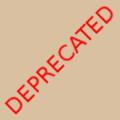CGAL 4.7 - dD Convex Hulls and Delaunay Triangulations
dD Convex Hulls and Delaunay Triangulations ReferenceSusan Hert and Michael Seel
This package provides functions for computing convex hulls and Delaunay triangulations in $$d$$-dimensional Euclidean space.

Introduced in: CGAL 2.3
BibTeX: cgal:hs-chdt3-15b
License: GPL
Deprecated:
This package is deprecated since the version 4.6 of CGAL. The package dD Triangulations should be used instead.

A subset $$S \subseteq \mathbb{R}^d$$ is convex if for any two points $$p$$ and $$q$$ in the set the line segment with endpoints $$p$$ and $$q$$ is contained in $$S$$. The convex hull of a set $$S$$ is the smallest convex set containing $$S$$. The convex hull of a set of points $$P$$ is a convex polytope with vertices in $$P$$.A point in $$P$$ is an extreme point (with respect to $$P$$) if it is a vertex of the convex hull of $$P$$.

CGAL provides functions for computing convex hulls in two, three and arbitrary dimensions as well as functions for testing if a given set of points in is strongly convex or not. This chapter describes the class available for arbitrary dimensions and its companion class for computing the nearest and furthest site Delaunay triangulation.

## Concepts

• ConvexHullTraits_d
• DelaunayLiftedTraits_d
• DelaunayTraits_d

## Classes

• CGAL::Convex_hull_d_traits_3<R>
• CGAL::Convex_hull_d<R>
• CGAL::Delaunay_d< R, Lifted_R >

Concepts

## Classes

class  CGAL::Convex_hull_d< R >

class  CGAL::Convex_hull_d_traits_3< R >

class  CGAL::Delaunay_d< R, Lifted_R >